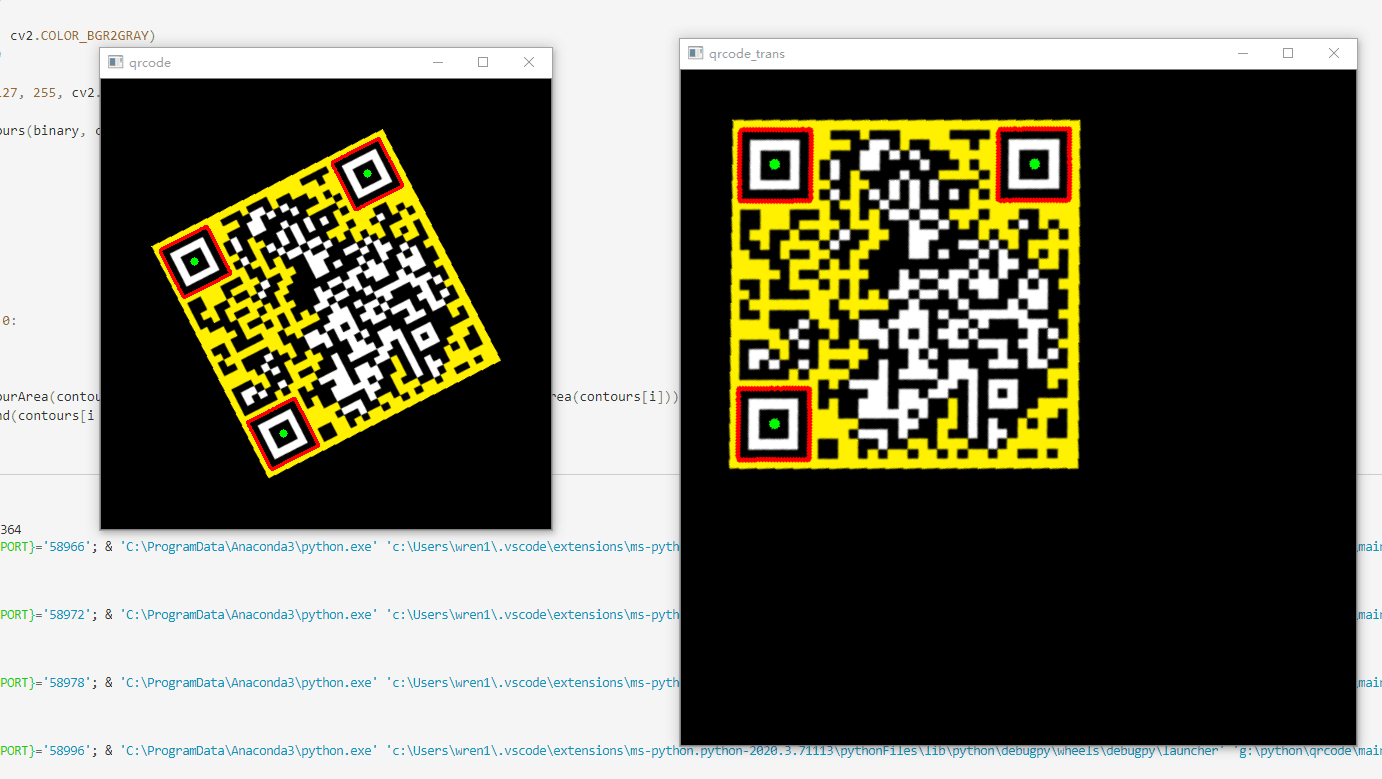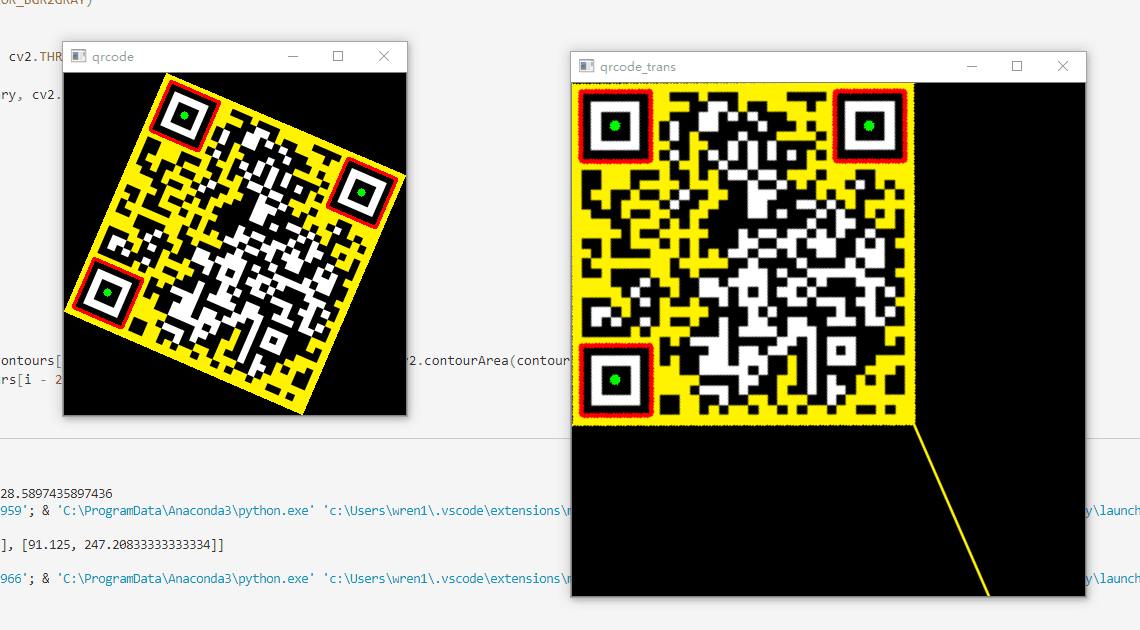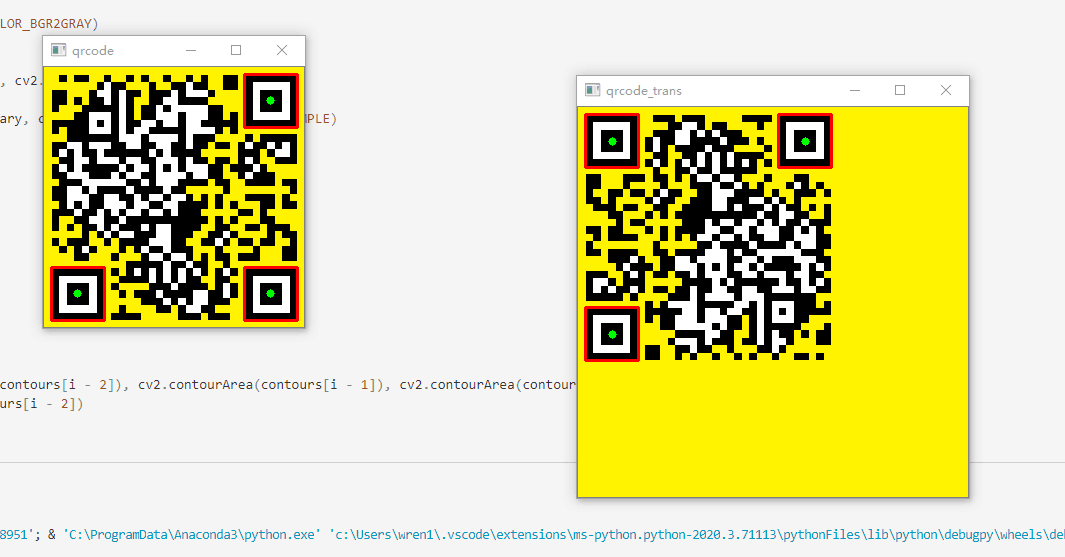## 效果展示## 代码说明

• 通过三层轮廓找到三个以上的疑似定位点
• 使用面积比确定大概率疑似定位点
• 使用判断三个点是否组成直角三角形判断是否是疑似定位点
• 通过规律和右手定则计算三个点的顺序（直角点为1，之后顺时针依次）
• 使用仿射变换将二维码矫正到标准姿态，以便后期读取。

## 代码详解

 123 import cv2 import numpy as np import math 

 12345678910 filename = 'qr.png' print("file:",filename) qrcode_input = cv2.imread(filename) qrcode = cv2.cvtColor(qrcode_input, cv2.COLOR_BGR2GRAY) # cv2.imshow("qrcode_gray", qrcode) _, binary = cv2.threshold(qrcode, 127, 255, cv2.THRESH_BINARY) contours = [] contours, hierarchy = cv2.findContours(binary, cv2.RETR_TREE, cv2.CHAIN_APPROX_SIMPLE) 

 123456789101112131415161718192021222324252627282930313233 def isQR_Point(con1, con2, con3): # // 57.46 base1 = con1 / 49 base2 = con2 / 25 base3 = con3 / 9 base = min(base1, base2, base3) if (base1 - base <= base / 5 and base2 - base <= base / 5 and base3 - base <= base / 5): return True return False level = 0 contours_sign = [] for i in range(len(hierarchy)): hie = hierarchy[i] if hie != -1: # 说明有下一级 level += 1 pass elif hie == -1 and level != 0: level += 1 if level == 3: # 找到了很有可能是的 if isQR_Point(cv2.contourArea(contours[i - 2]), cv2.contourArea(contours[i - 1]), cv2.contourArea(contours[i])): contours_sign.append(contours[i - 2]) pass pass level = 0 else: level = 0 pass pass cv2.drawContours(qrcode_input, contours_sign, -1, (0, 0, 255), 2) 

• 直角点为p1，之后顺时针依次为p2,p3的排列方式
 12345678910111213141516171819202122232425262728293031323334353637383940414243444546474849505152535455565758596061626364656667686970717273747576777879808182838485868788899091929394959697 ''' @name: 对点排序 @test: test font @msg: 直角为p1，顺时针依次为p2,p3 @param {type} @return: ''' def sort_Point(points): p1, p2, p3 = points # 勾股定理 很难计算范围 # 向量夹角 v12 = [p2 - p1, p2 - p1] # 向量1->2 v13 = [p3 - p1, p3 - p1] ''' @name: 计算两个向量夹角(数量积) @test: test font @msg: 使用公式cos_theta = (a * b) / (|a| * |b|) = (x1y1+x2y2)/(sqr(x1^2+y1^2) * sqr(x2^2+y2^2)) 欧几里德范数 @param {type} @return: ''' def get_rad(v1, v2): # print((v1 * v2 + v1 * v2) / (math.hypot(v1, v1) * math.hypot(v2, v2))) return math.acos( (v1 * v2 + v1 * v2) / (math.hypot(v1, v1) * math.hypot(v2, v2)) ) / math.pi * 180.0 pass # 判断三个角哪个是直角 ''' @name: 判断是否是这个范围内 @test: test font @msg: @param {type} @return: ''' def r_in(value, target=90, miss=10): if value > target + miss or value < target - miss: return False return True # print(p1,p2,p3) # print(v12,v13) if r_in(get_rad(v12, v13), 90): # 如果p1为直角，那需要继续判断p2,p3 if (v12 < 0 and v13 > 0) or (v12 < 0 and v13 < 0) or (v12 > 0 and v13 > 0) or (v12 > 0 and v13 < 0): # print(1) return [p1, p2, p3] elif (v12 > 0 and v13 < 0) or (v12 < 0 and v13 < 0) or (v12 > 0 and v13 > 0) or (v12 < 0 and v13 > 0): # print(2) return [p1, p3, p2] pass elif r_in(get_rad(v12, v13), 45): # 如果p1为45锐角，那可以判断p2,p3 if math.hypot(v12, v12) < math.hypot(v13, v13): # print(3) # 使用叉乘判断顺时针逆时针 右手定则 # 逆时针为[p2,p1,p3] # 顺时针为[p2,p3,p1] if np.cross(np.array(v12 + ), np.array(v13 + )) > 0: return [p2, p3, p1] else: return [p2, p1, p3] # print(np.cross(np.array(v12 + ), np.array(v13 + ))) else: # print(4) # print(np.cross(np.array(v12 + ), np.array(v13 + ))) if np.cross(np.array(v12 + ), np.array(v13 + )) < 0: return [p3, p2, p1] else: return [p3, p1, p2] pass return False # 求定位点中心点坐标 sign_point_center = [] for contour in contours_sign: # 每个中心点都要确认 # print(contour[:,0]) tmpcont = contour[:,0] # print(tmpcont.shape) avg_x, avg_y = 0, 0 for tmp_x, tmp_y in tmpcont: avg_x += tmp_x avg_y += tmp_y # print(tmp_x,tmp_y) avg_x /= contour.shape avg_y /= contour.shape # print(avg_x, avg_y) sign_point_center.append([avg_x,avg_y]) cv2.circle(qrcode_input, (int(avg_x), int(avg_y)), 4, (0, 255, 0), -1) pass # 对定位点排序，找到左上角1号点 # 分别为1号，2号，3号点 sign_point_center = sort_Point(sign_point_center) print(sign_point_center) 

 1234567891011121314151617181920212223242526272829 # 对图形进行仿射变换 min_x, max_x = min([x for x in sign_point_center]), max([x for x in sign_point_center]) min_y, max_y = min([x for x in sign_point_center]), max([x for x in sign_point_center]) if max_x - min_x > max_y - min_y: # 取长边 max_y = min_y + max_x - min_x else: max_x=min_x+max_y-min_y print(min_x, max_x, min_y, max_y) # M = cv2.getPerspectiveTransform( M = cv2.getAffineTransform( np.float32([ [sign_point_center, sign_point_center], [sign_point_center, sign_point_center], [sign_point_center, sign_point_center] ]), np.float32([ [min_x,min_y],[max_x,min_y],[min_x,max_y] ]) ) # qrcode_output = cv2.warpPerspective(qrcode_input, M, (qrcode_input.shape * 1, qrcode_input.shape * 1), flags=cv2.INTER_LINEAR, borderMode=cv2.BORDER_REPLICATE) qrcode_output = cv2.warpAffine(qrcode_input, M, (int(qrcode_input.shape * 1.5), int(qrcode_input.shape * 1.5)), flags=cv2.INTER_LINEAR, borderMode=cv2.BORDER_REPLICATE) cv2.circle(qrcode_output, (int(min_x), int(min_y)), 8, (0, 255, 0), 1) cv2.circle(qrcode_output, (int(max_x), int(min_y)), 8, (0, 255, 0), 1) cv2.circle(qrcode_output, (int(min_x), int(max_y)), 8, (0, 255, 0), 1) cv2.circle(qrcode_output, (int(max_x), int(max_y)), 8, (0, 255, 0), 1)